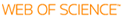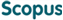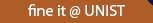### BROWSE

Multistage Shifted JACOBI Spectral Method for solving linear & nonlinear Fractional Differential Equations

Cited 0 times inCited 0 times inTitle
Multistage Shifted JACOBI Spectral Method for solving linear & nonlinear Fractional Differential Equations
Author
Issue Date
2015-02
Publisher
Abstract
In this work, we developed a new numerical method based on the shifted jacobi polynomials for solving linear and nonlinear initial value problem and boundary value problem of fractional differential equation. We extend the conventional spectral approaches such as the Shifted Jacobi Tau(SJT) method for linear problem and the Shifted Jacobi Collocation(SJC) method for nonlinear problem, by using the multistage methodology. These methods are called the Multistage Shifted Jacobi Tau(M-SJT) and the Multistage Shifted Jacobi Collocation(M-SJC) method,respectively. From the several illustrative examples, the advantages of using the proposed methods are discussed for the initial value problem and we compare the proposed methods with exact solution and conventional spectral approaches. In addition, we extend the proposed methods for solving nonlinear boundary value problem of the fractional differential equations. Since all proposed methods are developed for solving the linitial problem, it is necessary to convert the boundary problem to the initial problem. Here we adopt the nonlinear shooting method combined with M-SJC. From the numerical example, the advantages of using the proposed methods are discussed for the nonlinear boundary value problem and we compare the proposed methods with exact solution and conventional spectral approaches.
Description
Department of Mathematical Sciences
URIcan give you direct access to the published full text of this article. (UNISTARs only)